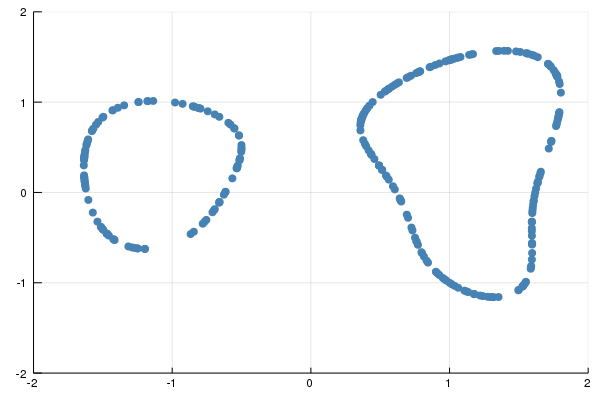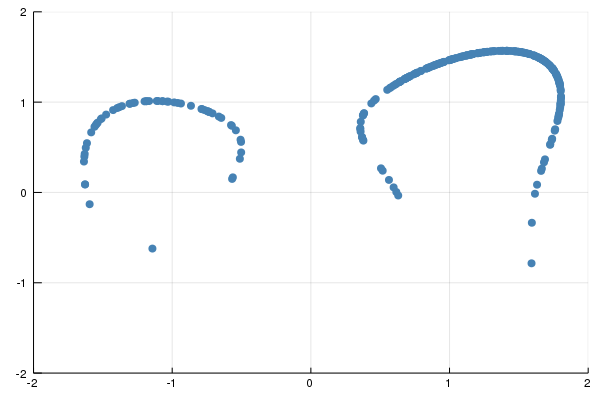# Sampling a probability distribution on a variety

By intersecting with linear spaces

The article Random points on an algebraic manifold proposes an algorithm for sampling from probability distributions on a variety based on linear slicing. In this example, we want to apply this algorithm to sample from the plane curve

$$V = \{ x=(x_1,x_2) \in \mathbb{R}^2 \mid x_1^4+x_2^4-3x_1^2-x_1x_2^2-x_2+1=0\}.$$

intersected with the box $B = [-2,2]\times [-2,2]$.

Suppose that $f:V\to \mathbb{R}_+$ is a measurable function. We want to sample from the density $f(x)/(\int_{V} f(x) \mathrm{d}V)$. We will consider the example $f(x)=1$, which gives the uniform distribution on $V\cap B$. For the algorithm we need upper bounds

$$K\geq \sup \{ f(x) \mid x\in V\cap B\} \quad \text{and}\quad C\geq \sup \{\Vert x\Vert^2 \mid x\in V\cap B\}.$$ We take $K = 1$ and $C = 8$.

The algorithm goes as follows. Intersect $V\cap B$ with a random line $L={a_1x+a_2y=b}$, where $a_1,a_2$ and $b$ are independent standard Gaussian random variables. Then, compute the following sum:

$$\overline{f}(a_1,a_2,b) := \sum_{x\in V\cap B\cap L} \frac{f(x)}{\alpha(x)}, \text{ where } \alpha(x)= \frac{1}{\pi} \frac{\sqrt{1+ \langle x,\pi_x x\rangle}}{1+\Vert x\Vert^2},$$

where $\pi_x$ is the orthogonal projection onto the normal space of $V$ at $x$. Because $d=\mathrm{deg}(V)=4$ has degree $4$, there are at most 4 points in the section $V\cap B\cap L$, and so

$$p:= \frac{\overline{f}(a_1,a_2,b)}{w} \leq 1, \text{ where } w=dK(1+C).$$

Then, we throw a biased coin $Z$ with $\mathrm{Prob}\{Z=1\} = p$.

• If $Z=1$, we choose $x\in V\cap B\cap L$ with probability $\frac{f(x) \alpha(x)}{\overline{f}(a_1,a_2,b)}$.
• If $Z=0$, we reject the sample.

This procedure produces points from the density $f(x)/(\int_V f(x)\mathrm{d}x)$.

Let us sample in Julia. First, we set up the equations

using HomotopyContinuation, LinearAlgebra, Distributions
@polyvar x[1:2]
F = x^4+x^4-3x^2-x*x^2-x+1
J = differentiate(F, x)
K, C, d = 1, 8, 4
w = d * K * (1+C)


and we define $\alpha(x)$.

function α(z, J)
N = normalize!([j(x=>z) for j in J])
sqrt(1 + (z ⋅ N)^2) / (1 + z⋅z) / pi
end


Now, we define a function that implements the rejection sampling.

f(x) = 1.0 # so that we sample the uniform distribution

function rejection_step(R)
if nreal(R) > 0
S = real_solutions(R)

# intersect M ∩ L with the box B
filter!(s -> abs(s) < 2 && abs(s) < 2, S)
if !isempty(S)
β = map(z -> f(z) / α(z, J), S)
f̄ = sum(β)
p = f̄ / w

# rejection step
if rand(Bernoulli(p)) == 1
p = β ./ f̄
i = rand(Categorical(p))
return S[i]
end
end
end
# return Inf if sample was rejected
return fill(Inf,2)
end


We create a suitable start system by intersecting $V$ with a random complex linear space.

@polyvar a[1:2] b
G = [F; a⋅x - b]
a₀, b₀ = randn(ComplexF64, 2), randn(ComplexF64)

start = solve([subs(g, a=>a₀, b=>b₀) for g in G])
start_sols = solutions(start)


Now, we track start_sols to various random real intersections, following this guide. In the code below we make $k=10^2$ trials to get points.

k = 10^2

#track towards k random linear spaces
points = solve(
G,
start_sols;
parameters = [a; b],
start_parameters =  [a₀; b₀],
target_parameters = [randn(3) for _ in 1:k],
transform_result = (R,p) -> rejection_step(R)
)
filter!(p -> !(Inf in p), points)


The points we get from executing this code are shown next.The code can easily be adapted to sample from other densities. For instance, changing to f(x) = exp(2x), K=exp(4) samples from a distribution that gives more weight to points in the upper half plane. Here is a picture.Cite this example:
@Misc{ sampling2022 ,
author =  { Paul Breiding },
title = { Sampling a probability distribution on a variety },
howpublished = { \url{ https://www.JuliaHomotopyContinuation.org/examples/sampling/ } },
note = { Accessed: June 27, 2022 }
}